# Recursive Function for Displaying a Linked List in C

## Recursive Function for Displaying a Linked List in C Language

In this article, I am going to discuss the Recursive Function for Displaying a Linked List in C Language with Examples. Please read our previous article, where we discussed How to Display Linked List in C Language with Examples.

##### Recursive Function for Displaying a Linked List in C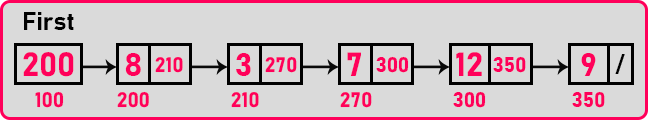This is a linked list that we want to traverse recursively. Let us write the function.

void Display(struct Node *p){
if(p! NULL){
printf(“%d”, p->data);
Display(p->next);
}
}

This is a recursive function. It takes a parameter of the type structure node pointer. Let us call the pointer name ‘p’. So, we have written its base condition and it can be continuation or termination. Here we have taken the condition for continuation. If ‘p’ is not null then we will print the p’s data that is data inside each node.

After printing data, it should go to the next node recursively so the display will call itself again bypassing the next node pointer that is Display(p->next)’. This function will display all the elements of a linked list one by one. So, it is printing and calling itself and moving on to the next node how it works. Now let us trace this function.

##### Tracing of Recursive Display Function for Displaying a Linked List:

We will call the function ‘Display (first)’,

###### Step1:

First, ‘d(200)’ will be called. We will write ‘Display’ as ‘d’. So,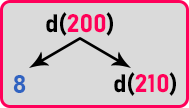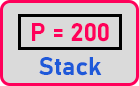After the call, the condition will be checked that is ‘if (p != NULL)’’. Here ‘p’ is not null, it is pointing to the node which has the address ‘200’. As the call is executed, so activation record will be created for this call ‘p = 200’. As the condition is true, it will 1st print the data of the node that is ‘8’ here. And next, it will call itself with a parameter ‘p->next’ means for the next node that is ‘d(210)’ here.

###### Step2:

Now, ‘d(210)’ will be called. So,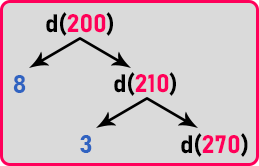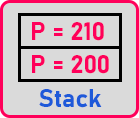Here also the condition will be checked that is ‘if (p != NULL)’’. Here ‘p’ is not null, it is pointing to the node which has the address ‘210’. As the call is executed, so activation record will be created for this call ‘p = 210’. As the condition is true, it will 1st print the data of the node that is ‘3’ here. And next, it will call itself with a parameter ‘p->next’ means for next node that is ‘d(270)’ here.

###### Step3:

Now, ‘d(270)’ will be called. So,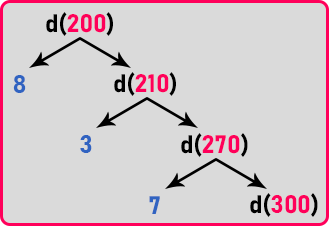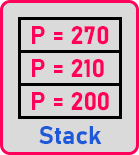Here also the condition will be checked that is ‘if (p != NULL)’’. Here ‘p’ is not null, it is pointing to the node which has the address ‘270’. As the call is executed, so activation record will be created for this call ‘p = 270’. As the condition is true, it will 1st print the data of the node that is ‘7’ here. And next, it will call itself with a parameter ‘p->next’ means for the next node that is ‘d(300)’ here.

###### Step4:

Now, ‘d(300)’ will be called. So,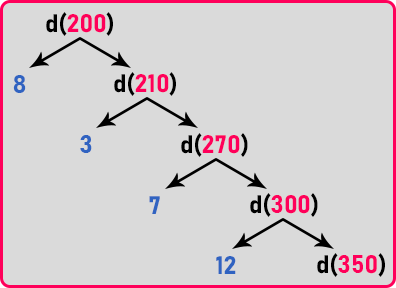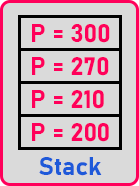Here also the condition will be checked that is ‘if (p != NULL)’’. Here ‘p’ is not null, it is pointing to the node which has the address ‘300’. As the call is executed, so activation record will be created for this call ‘p = 300’. As the condition is true, it will 1st print the data of the node that is ‘12’ here. And next, it will call itself with a parameter ‘p->next’ means for next node that is ‘d(350)’ here.

###### Step5:

Now, ‘d(350)’ will be called. So,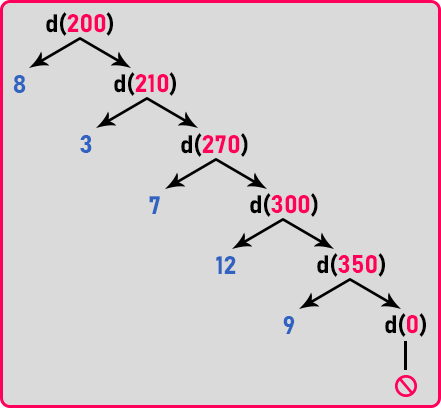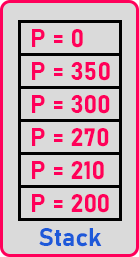Here also the condition will be checked that is ‘if (p != NULL)’’. Here ‘p’ is not null, it is pointing to the node which has the address ‘350’. As the call is executed, so activation record will be created for this call ‘p = 350’. As the condition is true, it will 1st print the data of the node that is ‘9’ here. And next, it will call itself with a parameter ‘p->next’ means for the next node that is ‘d(0)’ here.

Now we have traversed the whole linked list so there is no more node. So here ‘p’ will be null or ‘0’. This call will be executed and an activation record will be created ‘p = 0’ then the condition will be checked ‘if (p != NULL)’. Here ‘p’ is null so it will not enter in this ‘if’ block.

So here function will exit. This function will terminate this call ‘d(0)’ without doing anything. From here it will go back to its previous call that is ‘d(350)’. In this call also, all the task has been completed so control goes to the previous call ‘d(300)’ then ‘d(270)’, ‘d(200)’, ‘d(210)’ and last ‘d(200)’. So, all the calls will be terminated.

Also, the activation record will be deleted as the call terminates. So that’s all about the display function. Now let us analyze this recursive function.

##### Time Complexity:

We have already created a function using loop and. So how much time it will take. What is the work it is doing? Printing the data. Then printing takes how much time? Constant time for one node. Then how many times it is printing? Depends on the number of nodes. So, the time taken by the function is O(n). Whether the function contains a loop or it is a recursive function. It will take O (n).

##### Space Complexity:

The loop function is a simple function. There is no extra space is required. But recursive function uses the stack. So, it will take more space. And what is the size of the stack and the Total no. of calls that will be created? It is the number of nodes + 1. In this case ‘5 + 1’ is ‘6’. One for null also.

So, one more thing remembers if any procedure or a recursive function is written for traversing a linked list just once then it will be making ‘n + 1’ call for the entire linked list, and also the stack size will be endless ‘n + 1’.

Now we will make changes in this display function it is printing then calling itself recursively. We’ll just change the order first calling itself recursively then print. Then let us see what happens. Now, this is a different function.

void Display(struct Node *p){
if(p! NULL){
Display(p->next);
printf(“%d”, p->data);
}
}

So, the tracing tree for this one will be,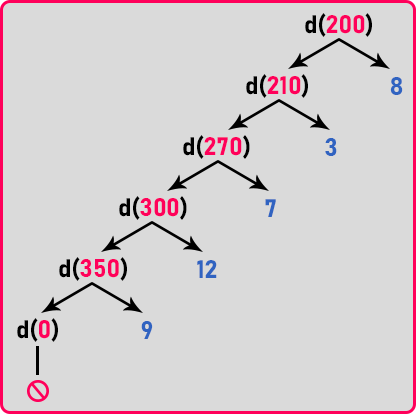We can see in the tree that the first function calls itself again and again till it terminates or the function base condition becomes false. When the function’s last call that is ‘d(0)’ will terminate then only printing will start means printing will be done at returning time of the function. And also, you can see in the tree diagram printing will be done in reverse order.

Output: 9 12 7 3 8

It will print the linked list in reverse order. So, the previous function prints the linked list in the same order, and this function will print the linked list in reverse order. So that’s all about traversing and displaying linked lists recursively. Now let us see the full code for this.

##### Program to Print Linked List in Same Order using Recursive Function in C:
```#include <stdio.h>
#include <stdlib.h>
struct Node
{
int data;
struct Node *next;
}   *first = NULL;

void create (int A[], int n)
{
int i;
struct Node *t, *last;
first = (struct Node *) malloc (sizeof (struct Node));
first->data = A;
first->next = NULL;
last = first;

for (i = 1; i < n; i++)
{
t = (struct Node *) malloc (sizeof (struct Node));
t->data = A[i];
t->next = NULL;
last->next = t;
last = t;
}
}

void RecursiveDisplay (struct Node *p)
{
if (p != NULL)
{
printf ("%d ", p->data);
RecursiveDisplay (p->next);
}
}

int main ()
{
struct Node *temp;
int A[] = { 3, 5, 7, 10, 25, 8, 32, 2 };
create (A, 8);
RecursiveDisplay (first);
return 0;
}
```
###### Output: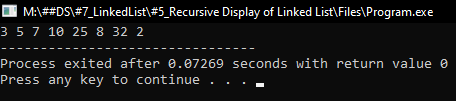##### Program to Print Linked List in Reverse Order using Recursive Function in C:
```#include <stdio.h>
#include <stdlib.h>
struct Node
{
int data;
struct Node *next;
}   *first = NULL;

void create (int A[], int n)
{
int i;
struct Node *t, *last;
first = (struct Node *) malloc (sizeof (struct Node));
first->data = A;
first->next = NULL;
last = first;

for (i = 1; i < n; i++)
{
t = (struct Node *) malloc (sizeof (struct Node));
t->data = A[i];
t->next = NULL;
last->next = t;
last = t;
}
}

void RecursiveRevDisplay (struct Node *p)
{
if (p != NULL)
{
RecursiveRevDisplay (p->next);
printf ("%d ", p->data);
}
}

int main ()
{
struct Node *temp;
int A[] = { 3, 5, 7, 10, 25, 8, 32, 2 };
create (A, 8);
RecursiveRevDisplay (first);
return 0;
}
```
###### Output: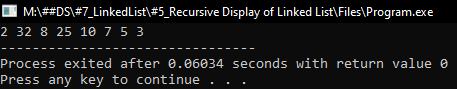In the next article, I am going to discuss Counting Nodes in a Linked List in C Language with Examples. Here, in this article, I try to explain the Recursive Function for Displaying a Linked List in C Language with Examples and I hope you enjoy this Recursive Function for Displaying a Linked List in C Language with Examples article.